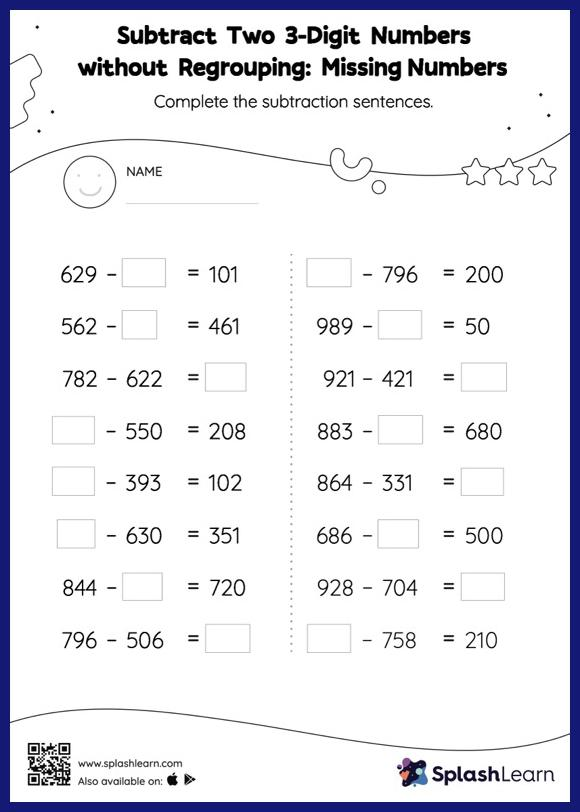# Subtract Two 3-Digit Numbers without Regrouping: Missing Numbers Worksheet

Home > Subtract Two 3-Digit Numbers without Regrouping: Missing NumbersTo find the missing number on this worksheet, students can utilize the count back strategy or the connection between addition and subtraction. In subtract two 3-digit numbers without regrouping worksheet, they do not need to regroup numbers to reach the solution. In this worksheet, students practice solving problems written in the horizontal format. How numbers are laid out in a problem affects the method a student employs to solve it. Therefore to develop actual fluency and mastery of multiple strategies, students must practice different formats.